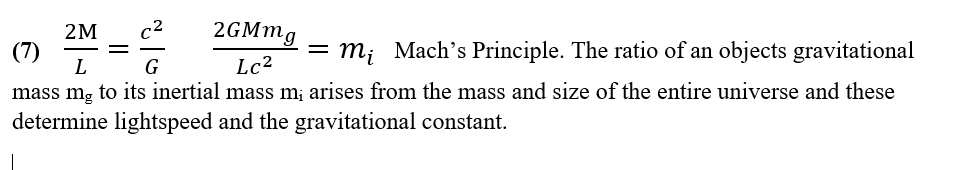Monday, 22 February 2021 17:04

## Equation 7Equation 7

Mach’s Principle has intrigued physicists for more than a century. It exists in a variety of formulations, most of them philosophical rather than mathematical.

Broadly stated Mach’s Principle, or the Einstein-Mach Principle, says that the inertia of any single body should depend on the mass and distribution of the whole of the rest of the universe.

Thus, the inertial mass of any body may not arise as an intrinsic property of that body but may depend on the entire universe.

In the Newtonian paradigm the inertial mass and the gravitational mass of a body always have the same ratio. Newtonian theory accepts this without explanation. In Einstein’s relativity the equivalence of inertial mass and gravitational mass becomes a central principle. The gravitational constant G represents the constant ratio between inertial and gravitational mass, but where does that come from?

In a universe whose size and density varies with time, any expression of Mach’s Principle becomes problematic, either the ratio of inertial mass to gravitational mass and hence G will vary with time, or lightspeed will vary with time, or both. We have no evidence of that having happened.

Only in a universe of constant size and constant overall density can Mach’s Principle apply and take mathematical form.

Equation 7 shows a mathematical expression of Mach’s Principle. It has no conceivable use, but it does look holistically and philosophically satisfying.